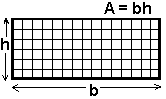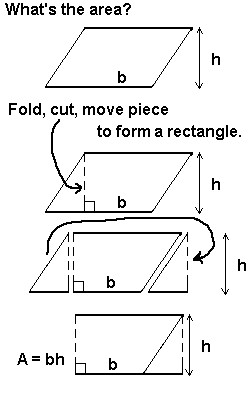Objective, Materials, Stuff To Review Before This Activities, Procedure, Rectangle, Parallelogram, Triangle, Trapezoid, Circle

 Objective To prove each of the area formulas by paper folding and/or cutting.

 Materials a pair of scissors, a ruler, a pencil, a sheet of rectangular paper for each of the following formulas: parallelogram, trapezoid, triangle, a waste paper bag or basket, a set of circluar papers for instructor use

 Stuff To Review Before This Activitiesdescriptions and vocabulary for the circle and each polygon, definition of area, area formula for a rectangle.       Point out that opposite sides of a rectangle are parallel.       Review at least as much about area as is discussed in rectangle, below.

 Procedure       Review area, area of a rectangle, properties of a rectangle such as parallel opposite sides and right angles.         These proofs need not be done at the same time. Each time one is done however, it is important to review the definition of area as the number of square units and to remind students that the reason "length times width" or "base times height" yields area is because it supplies the number of squares covered.         The rectangle must be discussed before the parallelogram.         The rectangle must be discussed before the triangle.         The rectangle and the parallelogram must be done before the trapezoid.

 Rectangle       Understanding why the area of a rectangle is base time height is the key to understanding each of these paper-folding proofs of area.Area means number of square units.         To compute the area, one must find the number of squares.         The base is the number of squares across the "bottom".         The height is the number of squares "high."         The base times the height is the number of squares, the area.

 Parallelogram Make the ParallelogramMake a parallelogram from a rectangular sheet of paper in the following way:   1st: On one edge, mark an arbitrary point A and draw a line segment to the indicated opposite vertex.   2nd: Repeat this on the opposite edge: mark point A on the edge parallel to the edge with A already marked on it so that the distance from the edges are the same. Again draw a line segment from A to the indicated opposite vertex.   3rd: Cut the paper on the line segment.   4th: Throw away the extra pieces of paper (the shaded triangles of the picture above).   Demonstrate A = bhTrapezoid Make the TrapezoidMake a trapezoid from a rectangular sheet of paper in the following way:   1st: On one edge, mark an arbitrary points A and B and draw line segments to indicated opposite vertices.   2nd: Cut the paper on the line segment.  3rd: Throw away the extra pieces of paper (the shaded triangles of the picture above).   Demonstrate A = (½)h(b1 + b2)Triangle### DON'T THROW ANYTHING AWAY!

Your goal is to create one "center triangle" (shown in white) and two other triangular pieces (shown in gray). You need each of these triangular pieces to prove the area of a (white) triangle is half the area of a (original piece of paper) rectangle with the same base and height.

1st: On one edge, mark an arbitrary point A and draw a line segments to each of the opposite vertices.

2nd: Cut the paper on the line segments. SAVE EACH OF THE THREE TRIANGULAR PIECES.

Demonstrate A = (½)bhThis page is brought to you by MATHEMATICAL CONCEPTS, inc., publishers of MATH SPOKEN HERE!, ISBN: 0-9623593-5-1.

You are hereby granted permission to make ONE printed copy of this page and its picture(s) for your PERSONAL and not-for-profit use. YOU MAY NOT MAKE ANY ADDITIONAL COPIES OF THIS PAGE, ITS PICTURE(S), ITS SOUND CLIP(S), OR ITS ANIMATED GIFS WITHOUT PERMISSION FROM: sb@mathnstuff.com or by mail to MATHEMATICAL CONCEPTS, inc., 85 First Street, Keyport, NJ 07735.© 3/2003, 7/2020, A2 www.mathnstuff.com/math/spoken/here/2class/150/area.htm